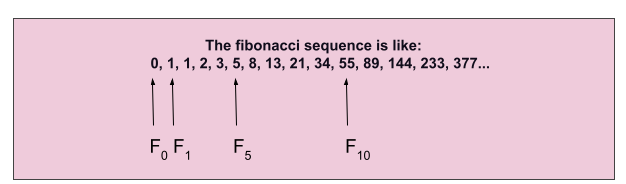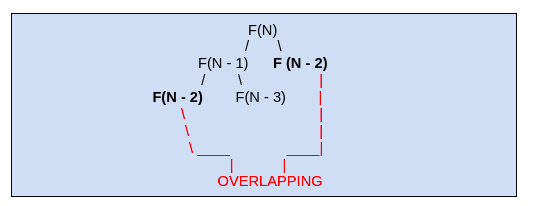# Fibonacci numbers

### Introduction

The fibonacci series is the number sequence in which the given number results from adding the two previous numbers. The terms in the Fibonacci sequence are as follows:The individual numbers in this sequence are called Fibonacci numbers. The Fibonacci sequence is a fantastic mathematical concept found in various places, including seashell patterns and the Parthenon.

#### Properties of Fibonacci Numbers

FN + K = FK . FN + 1 + FK - 1 . FN

• Applying Addition Rule to the case, K = N

FN + N = FN . FN + 1 + FN - 1 . FN

F2N = FN ( FN + 1 + FN - 1)

• From the above property, we can prove that for any positive integer K, FNK is the multiple of F(By the Induction Hypothesis).
• The inverse of the above property is true since, if FM is multiple of FN, then M is also the multiple of N.
• Cassini’s Identity

FN - 1 . FN + 1 - FN2 = (-1)N

This identity was given by Giovanni Domenico Cassini, an Italian mathematician. In the mathematical expression, N is the variable and it can have values from 1…..N.

For eg., if ‘N’ is an odd number, i.e. 1, 3, 5,... then (-1)N+1 = +1 in every case. But if we take even value here, we will get the result as -1.

• GCD Identity

For M, N >= 1

GCD(FM , FN) = FGCD (M, N) , where M, N are integers

### The Golden Ratio Approach

The golden ratio is defined as the limit of the ratio of successive terms in the Fibonacci sequence.

The golden ratio is coming about 1.618, as it means that as ‘N’ becomes sufficiently large, the fibonacci sequence approaches or approximates a geometric sequence. So, starting at number 144, and if we multiply 144 by 1.618, we get 233 (Approx.), the next element in the sequence. If we now multiply 233 with 1.618, we get approximately 377. Hence this golden ratio helps to approximate the next number in the series easily.

The formula for golden ratio is,

For example, let us find out the 8th element in the sequence using the formula,

(1 + √5) - (1 - √5)8

F8 = ------------------------------ = 21

28 . √5

### Code:

Output:

The matrix multiplication in O(log N)

### Code of Fibonacci Number Matrix Multiplication in C++

Input:

Output:

#### Time Complexity

The time complexity depends on two factors:

• Matrix multiplication, K3

As the matrix moves ‘column by column’ or ‘row by row’, we will get a matrix K x K where ‘K’ represents the number of states. We can notice that in our code, the function ‘multiply’ has three nested for loops which takes time complexity = K x K x K =  K3.

• Log(N)

For computing the large input, we raise the matrix to the power N, which takes log(N) time.

Hence, multiplying both the factors (1) and (2), we get => K3. log(N)

Therefore, the time complexity will be O(K3 log N).

#### Space Complexity

The space complexity is O(log N) since we raise the matrix to the Nth power. Hence it will take O(log N) additional space.

What is the golden ratio in the Fibonacci sequence?

From atoms to the massive stars in the sky has patterns. The golden ratio reveals predictable or observable patterns. This ratio is derived from the Fibonacci sequence, named after Leonardo Fibonacci, an Italian mathematician.

What is the time complexity of finding the Nth fibonacci term by recursion?

The time complexity by recursion is O(2N).What is the time complexity of finding Nth fibonacci term with DP?

For finding the Nth fibonacci number, we got O(N) time complexity in the dynamic programming method.Here, we store the previous terms, which are overlapping to reduce the time complexity.

### Key Takeaways

In this blog, we learned about Fibonacci numbers, their properties, the working of matrix multiplication in O(log N) time complexity, and the golden ratio approach.

Try problems related to the Fibonacci numbers here on CodeStudio to understand the working concept of Fibonacci numbers. To be more confident in data structures and algorithms, try out our DS and Algo Course.CREDITS: GIPHY

By Sneha Mallik